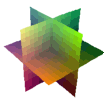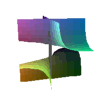### Unfoldings

Back

These can be formed by taking a normal singularity and adding some lower degree terms.

#### A1--A1--

x^2-y^2-z^2Hyperboloid of 1 sheet

x^2-y^2-z^2 =-0.1Hyperboloid of 2 sheet

x^2-y^2-z^2 =+0.1

#### D4--D4--

x^2 y-y^3-z^2One A3

x^2 y-y^3-z^2 - a x^23 A1

x^2 y-y^3-z^2 - a(x^2-y^2)3 A1

x^2 y-y^3-z^2 + a(x^2-y^2)

#### Three PlanesThree planes

x y z=0Two crosscaps

(Crosscap propeller)
x y z + a(x^2+y^2)=0Cone and a plane

x y z + x^3